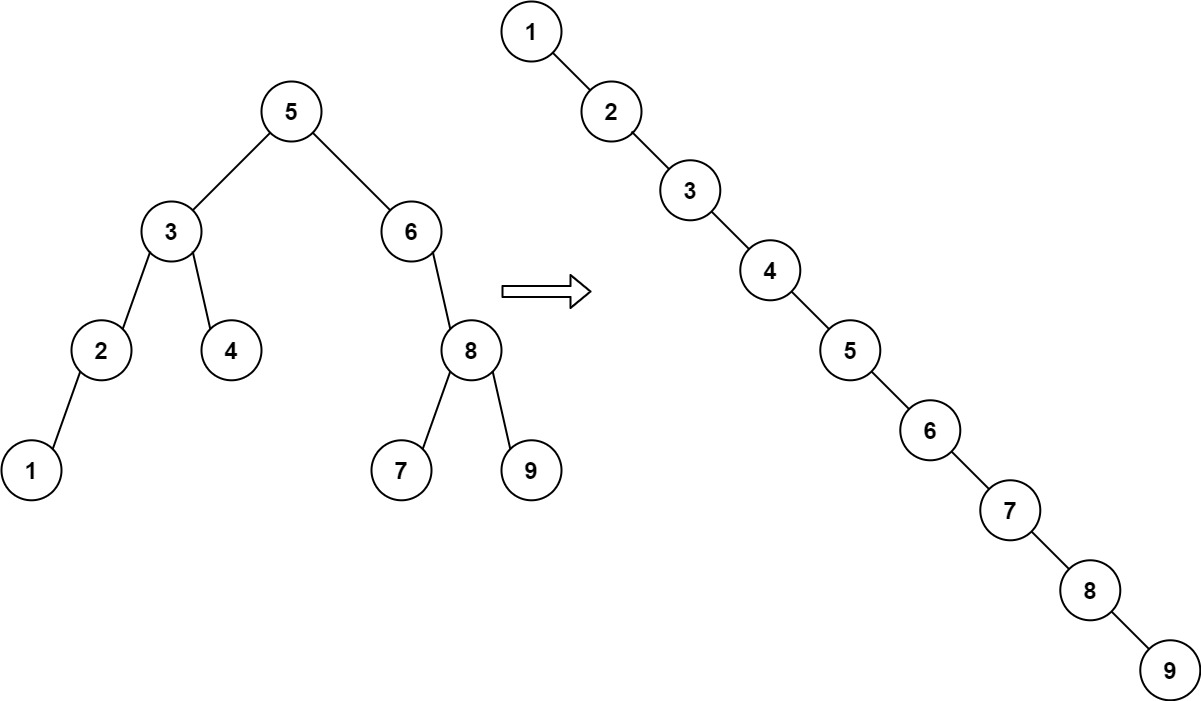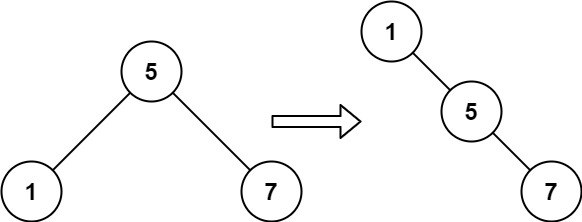# 897. Increasing Order Search Tree

Increasing Order Search Tree - LeetCode

Given the root of a binary search tree, rearrange the tree in in-order so that the leftmost node in the tree is now the root of the tree, and every node has no left child and only one right child.

Example 1:Input: root = [5,3,6,2,4,null,8,1,null,null,null,7,9] Output: [1,null,2,null,3,null,4,null,5,null,6,null,7,null,8,null,9] Example 2:Input: root = [5,1,7] Output: [1,null,5,null,7]

Constraints:

``````The number of nodes in the given tree will be in the range [1, 100].
0 <= Node.val <= 1000
``````

• code O(N), O(N)
``````class Solution:
def increasingBST(self, root: TreeNode) -> TreeNode:
self.flat = []
def inorder(node):
if not node: return
inorder(node.left)
self.flat.append(node)
inorder(node.right)
inorder(root)
for i in range(len(self.flat)-1):
self.flat[i].left = None
self.flat[i].right = self.flat[i+1]
self.flat[-1].left = None
self.flat[-1].right = None
return self.flat
``````
• code O(N), O(H) space, where H is the height of the given tree, and the size of the implicit call stack in our in-order traversal.
``````class Solution:
def increasingBST(self, root):
def inorder(node):
if node:
inorder(node.left)
node.left = None
self.cur.right = node
self.cur = node
inorder(node.right)

ans = self.cur = TreeNode(None)
inorder(root)
return ans.right
``````